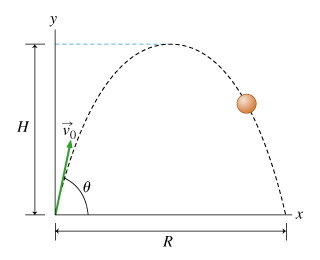# Problem: a) Find the time that it takes the projectile to reach its maximum height H. Express the answer in terms of v0, theta, and g (the magnitude of the acceleration due to gravity). b) Find tR, the time at which the projectile hits the ground after having traveled through a horizontal distance R. Express the time in terms of v0, theta, and g.

###### FREE Expert Solution

a)

Using the kinematic equation:

$\overline{){{\mathbf{v}}}_{{\mathbf{f}}}{\mathbf{=}}{{\mathbf{v}}}_{{\mathbf{0}}}{\mathbf{+}}{\mathbf{a}}{\mathbf{t}}}$

vfy = 0, v0y = v0 sin θ, and a = -g

80% (40 ratings)###### Problem Detailsa) Find the time that it takes the projectile to reach its maximum height H. Express the answer in terms of v0, theta, and g (the magnitude of the acceleration due to gravity).

b) Find tR, the time at which the projectile hits the ground after having traveled through a horizontal distance R. Express the time in terms of v0, theta, and g.